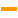# Subtract

 Block Group: Math Operations Icon:The Subtract block subtracts its arguments.

Subtraction is calculated in order, top to bottom.

For information on using dataflow blocks, see Dataflow.

For accepted and excluded arguments of Math Operations blocks, see Math Operations.

## Input/Output Property

The following property of the Subtract block can take input and give output.

• input n (number)

input n specifies one of the numbers to subtract.

## Output Property

The following property of the Subtract block can give output but cannot take input.

• output (number)

output returns the result of subtracting all of the input values.

## Examples

The following image shows two examples of the Subtract block. In the rightmost Subtract block, two different data types are used: a number and a string representation of a number.